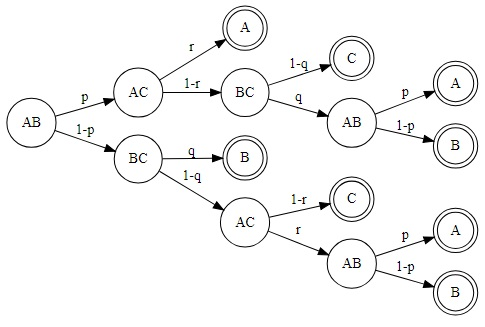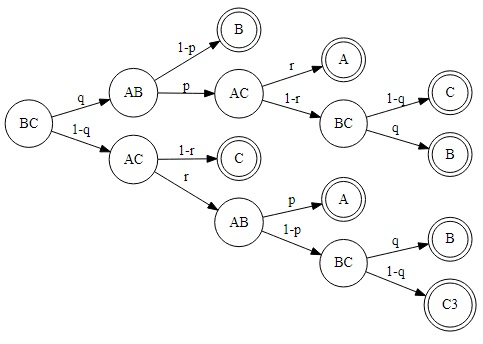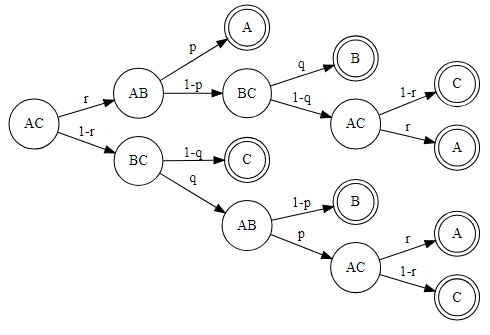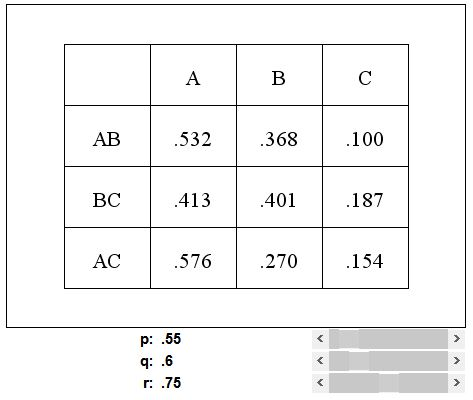# Chess Players Truel

Players A, B, and C play a series of chess games. Assume that A is the strongest player and C is the weakest one. Assume that there is no tie for each game. The winner of each game will play with the 3rd player. The player who first gets 2 wins is the winner of the series. The player B determines who will play the 1st game. Find the best choice for B. In general: if probability of A to win B is p > .5, probability B to win C is q > .5, probability A to win C is r, and r > p, evaluate chances of B.

SolutionPlayers A, B, and C play a series of chess games. Assume that A is the strongest player and C is the weakest one. Assume that there is no tie for each game. The winner of each game will play with the 3rd player. The player who first gets 2 wins is the winner of the series. The player B determines who will play the 1st game. Find the best choice for B. In general: if probability of A to win B is p > .5, probability B to win C is q > .5, probability A to win C is r, and r > p, evaluate chances of B.

The simplest way to approach the problem is by constructing an event tree. We are going to have three of them. The trees grow downwards starting with one of the pairs AB, BC or AC. Each node is a game, except for the terminal ones shown in double circles. These are the winners of the tournament who collected two wins first. For the first two trees we'll find the probability of B being the winner. These will be denoted PAB and PBC. We assume the outcomes of all games are independent.PAB = p·(1 - r)·q·(1 - p) + (1 - p)·q + (1 - p)·(1 - q)·r·(1 - p).PBC = q·(1 - p) + q·p·(1 - r)·q + (1 - q)·r·(1 - p)·q.The task is to compare the two expressions PAB and PBC assuming p, q, r > .5 and r > p. You can play with the applet below to verify that always PAB < PBC which we shall prove below. The result is imminently plausible as it seems quite reasonable for B to start with playing the weakest player (C) first.

### If you are reading this, your browser is not set to run Java applets. Try IE11 or Safari and declare the site https://www.cut-the-knot.org as trusted in the Java setup.What if applet does not run?

So here are the two expressions to compare:

1. PAB = p·(1 - r)·q·(1 - p) + (1 - p)·q + (1 - p)·(1 - q)·r·(1 - p).
2. PBC = q·(1 - p) + q·p·(1 - r)·q + (1 - q)·r·(1 - p)·q.

In the difference PBC - PAB, the common term (1 - p)·q cancels out leading to

PBC - PAB= [p·q·(1 - r) + (1 - p)·(1 - q)·r]·(p + q - 1)

which, since p + q > .5 + .5 = 1, is positive. Note that this is true even without the condition r > p.